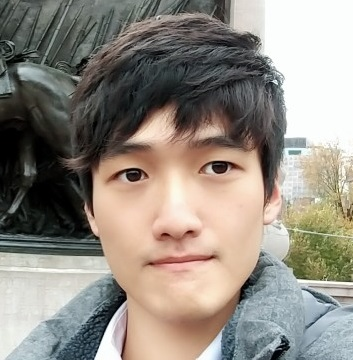Jaewoo Song

### Categories

• Tech

Beam Search is a tree search algorithm based on “Best First Search” method used in various NLP tasks frequently.

As we can see from its name, Best First Search algorithm is a method that explores a graph by searching the most promising node based on certain rules, which is heuristic.

Likewise in Beam Search, we conduct decoding process searching through sequences while keeping the several best options.

This makes up for shortcomings of original decoding procedures in NLG tasks in seq2seq or transformer architectures.

The limitations of greedy decoding

The decoder in original seq2seq or transformer models makes the output sequence in greedy method.

In other words, we just select the most probable word at each time $t$, only considering previous tokens generated, not future candidates.

So we make the best decision in the current certain moment.

But this can lead to unwanted or defected outputs since the decoder does not find the most optimal result among all possible combinations.

This is a major problem not only in this case but also in usages of a greedy algorithm itself.

So we always need to verify that the greedy method we are using eventually gives us the optimal answer, but unfortunately the decoder in above models does not.

Let’s see an example.

Assume that we are going to make a sentence “I enjoy playing games with the video game console.”As we can see, the decoder predicts exactly one word at a certain time $t$.

But we cannot assure that this “one word” is the best choice, which can be a unwanted token with slightly better probability than that of original desired one.

In above example, there is no problem that ‘the’ comes right after ‘playing’ since this does not destroy the overall structures or meanings of the sentence at this point.

So the model might choose ‘the’ instead of ‘games’.

After choosing it, we cannot undo the choice and this error is passed to next phases on and on.

Eventually, the whole sequence is defected only due to this first false choice.

Before going into the details of beam search, let’s check one simple naive solution.

How about checking all combinations of words to find an optimal result?

Needless to say, this is too time consuming since if we say that the total number of words is $V$ and the maximum length of sequences is $L$, then the time complexity becomes $O(V^L)$.

Obviously this takes too long so the beam search can be a reasonable option.

Application of the beam search

What we’re gonna do now is that keeping a few sequences with high probability to prevent one mistake from corrupting the entire output.

In other words, since we cannot check all combinations, a compromise can be searching the best result among selected several sequences.

Let’s say that the beam size is $k$, which is the number of branches we are going to keep.

The process of the beam search is as follows.

1. When decoding a token from previous completed sequence, take top $k$ words with higher probability.
2. Expanding all branches possible, choose top $k$ branches considering each total score.
3. Keep conducting the decoding steps with chosen $k$ branches.

For example, if we set $k=2$, then the whole procedure goes like below description.The figure is quite clumsy, but I think this will help you to understand how the beam search works.

And many people might be curious about several things, so we will look into it from now on.

First, the beam search does not guarantee the optimal answer, as you can see.

It just expands the search space so that we don’t miss possible better sequences, but it does not make us consider all combinations.

But it is certainly more effective than exhaustive search and gives us a quite better result than original greedy method does.

Second, it is not certain to decide when to stop decoding in beam search.

In greedy search, we just have to stop once the end token comes out, but the beam search has many hypotheses that have different termination timings.

One way is to set desired sequence number $n$.

If we meet the end token in one branch, then we just keep this sequence and continue to explore another branches using beam search.

Once the number of kept results become $n$, then we stop decoding and choose the best sequence.

Another way is to set a hyperparameter $T$ which specifies the certain time step to stop.

So we just keep decoding until the step reaches $T$, then get decoded sequences.

After that We can get the best result from our candidate results.

But there is one problem.

Sequentially choosing one word can be considered as multiplying each conditional probability to total score. (Or adding each log value to total log probability.)

Since the probability is between $0$ and $1$, choosing more words makes the total score smaller.

In other words, the longer the sentence is, the more disadvantageous it is to be chosen, which is not fair.

So there is a way to normalize scores by the length as follows.

$\frac{1}{t}\sum^{t}_{1}logP(y_i \mid y_1, y_2, ... y_{i-1}, x)$

So this is about beam search so far.

It is common method to generate a better result in many NLG tasks, such as Neural Machine Translation, Dialogue system, Text summarization, etc.

Although it is a bit complicated to implement into codes, it is certainly worth knowing.

Lecture 8: Machine Translation, Sequence-to-sequence and Attention . (2020, Jan 30). http://web.stanford.edu/class/cs224n/slides/cs224n-2020-lecture08-nmt.pdf.
Lecture 15: Natural Language Generation . (2020, Feb 25). http://web.stanford.edu/class/cs224n/slides/cs224n-2020-lecture15-nlg.pdf.